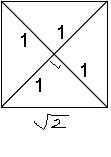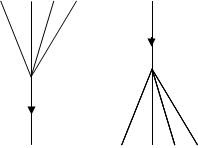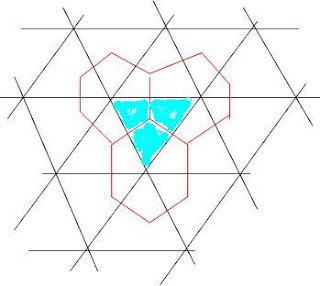## Blogrolling On

The consistently intriguing posts of The Everything Seminar has landed it on the sidebar. The latest post about summing divergent series includes the generating function

$T(z) = 1 + z + 2 z^2 + 5 z^3 + 14 z^4 + 42 z^5 + \cdots$

for the Catalan numbers. M theorists will recognise the coefficients as the number of vertices on an associahedron: two vertices for a line segment, five for a pentagon, and so on. And lest there be any doubt he is thinking about trees, he refers to this paper, which maps 7-tuples of (planar rooted binary) trees to trees. A commenter (with a blog called God Plays Dice) pointed out that a different set of trees gave another isomorphism between 5-tuples and singlets.

Apparently the reason the number 7 works for general trees is because 7 = 6 mod 1 and by dividing trees into left and right halves we get an equation $T = 1 + T^2$, where the 1 stands for the root. So planar rooted trees are associated to a sixth root of unity, and the fourth root case is about trees with either 0, 1 or 2 children at each vertex. Its generating function $M$ yields the series

$M(1) = 1 + 2 + 4 + 9 + 21 + 51 + · · · = −i$

It seems there are lots of cute ways of writing down complex numbers as infinite sums, so long as one uses series derived from trees! Here’s a cool TWF on this stuff, with a link to this helpful, and seminal, paper by Fiore and Leinster. Oh, I can’t wait to go and play some more…

## Solar Superorbits

At last, a new paper by A. L. Kholodenko on the Quantum Signatures of Solar System Dynamics. From the abstract:

“In this work new uses of Heisenberg’s ideas are found. When superimposed with the equivalence principle of general relativity, they lead to quantum mechanical treatment of observed resonances in the Solar system. To test correctness of our theoretical predictions the number of allowed stable orbits for planets and for equatorial stable orbits of satellites of heavy planets is calculated resulting in surprisingly good agreement with observational data.”

The first part of the paper is an excellent outline of the modern form of Heisenberg’s honeycomb quantum mechanics along with an historical account of its motivation. Happy reading.

## Tour De Force

Warning: make sure you are sitting down before you attempt to look at this 642 page draft of the new book, Noncommutative Geometry, Quantum Fields and Motives, by Matilde Marcolli and Alain Connes.

Not content with introducing QFT, NCG, Connes’ Standard Model, the Riemann hypothesis, motives and the kitchen sink, they finish up (from page 611) with a section entitled The analogy between QG and RH. The preface makes it abundantly clear that the book is primarily about this, as yet mysterious, correspondence. Unfortunately, I suspect I will find most of the book extremely mysterious as long as I live.

## M Theory Lesson 79

Event Symmetry has also long pondered the j-invariant, and now has a post relating this invariant to a hyperdeterminant of type 4, which is to say a function of a 2x2x2x2 hypermatrix. He points out that the degree of the polynomial is the magical number 24, the dimension of the Leech lattice. Note that Michael Rios has also been keen on hyperdeterminants for some time because of their appearance in the quantum computational approach to Black Hole entropy.

## M Theory Lesson 78

The last Riemann post raised again the issue of defining numbers in terms of diagrams. In logos theory one cannot simply pull infinite sets, or real numbers, out of a hat. The rabbit prefers to live in a burrow, where any number represents an enormous variety of objects.

The Euler characteristic of a category was one natural way of assigning rational numbers to finite type diagrams. What about irrational numbers? Legend has it that Pythagoras treated Hippasus rather badly (perhaps by killing him) after Hippasus demonstrated that the square root of 2 was irrational.A proof begins by assuming that $\sqrt{2}$ is rational and hence expressible as an indecomposable ratio of two integers $a$ and $b$, and then derives a contradiction. This relies on the concept of primeness for the ordinals. From the classical assumption that, if P is false, (not P) must be true it is deduced that $\sqrt{2}$ is irrational, the only alternative to being rational. Ah, hang on a minute. In logos theory we don’t want to assume that complement is an involution, so the proof doesn’t quite work, but there clearly needs to be more than one kind of number. The geometric definition of $\sqrt{2}$ uses two squares of side length 1, which are both cut in half and glued together as shown. This assumes that the correct units for area are $L^{2} = L.L$ where $L$ is a unit of length, but of course $L$ could be any unit. It seems there are an awful lot of assumptions being made to define irrational numbers. In three dimensions in M Theory the complement is a triality. Thus we should probably assign a different numerical status to $\sqrt{2}$ and $\sqrt{3}$. And only when we get to weak $\omega$ categories do we get all the reals.

If we tried to measure a real edge of a square of unit area we could never show it was exactly $\sqrt{2}$ because that would require subdividing the interval further and further until the atomic, or subatomic, structure of the environment altered our sense of the edge, or altered the edge itself so that there was no longer anything to measure.

On the other hand, zeta values show up as soon as one starts thinking about knots and QFT, although the zeta values are treated as abstract basis elements for an algebra over the rational numbers. It is convenient to assign real number values to them in order to evaluate physical quantities, but this comes from the rules of ordinary complex analysis, which we would like to move beyond.

## M Theory Lesson 77

Posts here often discuss operads, for which compositions are represented by a directed rooted tree and the root is the single output. Dually one can consider cooperads with multiple outputs.These give rise naturally to coalgebras rather than algebras. And yes, people have considered vertex operator coalgebras and so on. Recall that coalgebras are essential to the concept of Hopf algebra. An example of a Hopf algebra is a universal enveloping algebra of a semisimple Lie algebra. Deformations of these, also Hopf algebras, are the notorious quantum groups.

Now Ross Street has published a book, Quantum Groups: a Path to Current Algebra, which I would love to get my hands on. The book blurb says, “A key to understanding these new developments is categorical duality.” That simply means the duality given by turning a tree upside down, as drawn, so we need operad structures that combine upward branches and downward branches. Leaping ahead to triality, one expects branchings in three directions and no chosen root. Such diagrams are associated to cyclic operads, and we actually consider these with polygonal tilings, since the dual to a polygon is a branching tree with no real root.

## Higgs Blogging II

The Higgs mechanism is without a doubt one of the most beautiful features of the Standard Model. This may, however, be an argument in favour of considering it a natural effective mass generator, rather than an argument for taking its particle nature seriously. Mathematicians such as N. Hitchin have run with Higgs fields and constructed fantastic spaces. Alain Connes has even reformulated the Standard Model using NCG. But again, this seems to be an argument in favour of the Higgs being a stopgap for something deeper. For those of us who work on a QFT not based on symmetry or Lagrangians, the Higgs can only potentially exist as a derived concept, in which case there is no need to posit it a priori.

But the biggest problem with the Higgs is its attachment to the vacuum, and its pervasion of space. That’s simply not something that particles do. So just because nobody has yet fully explained the particle masses any other way, doesn’t mean the Higgs boson exists. How can we possibly hope to understand the Higgs mechanism without understanding mass?

A guest poster on The Everything Seminar today points out that most of our baryonic mass comes from the binding of quarks and other partons in atomic nuclei, and not from the rest mass of quarks themselves. In other words, an unseen seething relativistic swamp of matter, for which we don’t even have a real theory, makes up most of the mass we care about, unless we want to consider Dark Matter as well, in which case we clearly don’t know very much about anything. This is the realm of quantum gravity, not the Standard Model. Assuming Dark Matter requires a whole new category of observables, obliterating our trust in the old ones, and leads to the expectation of new physics at the TeV scale.

## Higgs Blogging

While checking out the latest New York Times story on the fairy field, I found an anti-establishment physics blog that’s way more interesting than Not Even Wrong: check out this blog by R. Mirman, complete with links to error messages for the cosmological constant. This old guy is angry.

Clifford seemed a little bothered by my continued vocal disbelief in the existence of fairy fields, and he said, “Hi Kea! I see you’re still ahead of us fools who wait for experiments before concluding what we believe about Nature.”

Well, actually, as it happens, I was, and still am, of the opinion that theoretical science is at least partly about trying to understand the outcomes of experiments before they are performed. It is therefore my duty, as a professional (albeit unpaid) quantum gravity theorist, to make sensible predictions about events at the LHC, and elsewhere. And then there is the not so minor issue of all the previous experiments that were supposed to see certain fairy particles, but didn’t. What are all those experimentalists doing, anyway?

Meanwhile Amanda, at Tommaso Dorigo’s blog, said (in disparagement as usual), “Almost as bad: Famous Physicist says: Here is a really interesting idea: let’s all use category theory/twistors/moonshine/-fill in badly motivated or frankly unphysical idea which is clearly going nowhere HERE-.”

Wow! Isn’t that cool! Category Theory and Twistors and Moonshine are getting mocked all in one breath! Most of the physicists I know still haven’t thought about these things. Maybe physics is progressing as it should, after all.

So why does the Higgs not exist? For starters, there never was any reason to think it should. As everyone knows, QFT likes particles to be massless. QFT does not solve mass generation: it includes masses as parameters. Secondly, our best bets for observables in a theory which does actually describe mass, doesn’t include in any obvious way a Higgs particle. In other words, it doesn’t exist. Other stuff probably exists, but it’s different stuff. Now many people keep insisting on telling me that physical observables are about symmetry, and this friendly advice is usually accompanied by much hand-waving about Lagrangians and representation theory and other mathematics from QFT, which never had anything to do with gravity, as it is observed.

By the way, those infernal twistors also say interesting things about massless particles, like about how they might be viewed in a unified setting using cohomology. And like how higher non-Abelian cohomology is needed to understand mass. Now I realise that QFT doesn’t usually deal with twistors, but Nature (the bitch) isn’t going to let QFT reign supreme forever, folks, especially when it comes to the issue of mass generation.

It seems obvious that the fairy field is unlikely to exist, even without formally extending the notion of observable into the language of motivic cohomology and moonshine (but that sounds like fun). Maybe I’m just stupid and I never understood QFT and there really is a Higgs, which Fermilab will find any day now. I guess we’ll wait and see, just like we’ll keep waiting for protons to decay, or gravitational waves to magically appear in the M31 GRB event.

Update: Tony Smith just posted an article on moonshine monsters, Jordan octonions, and the Standard Model here. Enjoy.

## M Theory Lesson 76

Recall that Postnikov computed the volumes and number of lattice points for general permutohedra in a variety of ways. In particular, volumes for standard permutohedra can be computed using Weyl group lattices. For $S_{3}$ in dimension 2 one compares the area of 6 (the order of the group) triangles, such as the blue triangle shown, to the sum of the areas of the three hexagons (permutohedra).The hexagons are drawn via a specific algorithm. First pick a point in the blue triangle, the central vertex. This point has an image in the other triangles. The convex hulls of these points give the collection of hexagons. Note that these hexagons look like the central piece of a honeycomb for 3×3 matrices. Sorry about the wonky picture.

## Riemann Rocks

If we don’t worry about complex numbers, various forms of the Riemann Hypothesis have known to be true for some time now. Bombieri came up with a simple proof for algebraic curves over finite fields  in the early 1970s.

Let $k$ be the finite field with $q$ elements and $C$ a curve over this field. The zeta function is about divisors on $C$, which we can think of as formal collections of points on the curve. Prime divisors will be labelled $P$. Now let $d(P) = [k_{P} : k]$, which is the degree of a field extension $k_{P}$, the so-called residue field. The variables $t$ and $s$ will be used, where $t = q^{-s}$. The Euler product formula for the zeta function takes the form

$\sum_{D} N(D)^{-s} = \prod_{P} (1 – N(P)^{-s})^{-1}$

where the sum is over divisors and the product over prime divisors, and $N(P) = q^{d(P)}$. So it looks just like the usual Riemann zeta formula, except that numbers have been replaced by (numbers associated to) geometric objects. If we let

$L(t) = \zeta (t) (1 – t) (1 – qt)$

and let $a_{i}$ be the inverses of the roots of $L(t)$, then the Riemann hypothesis has the following simple form: the $a_{i}$ all satisfy $| a_{i} | = \sqrt{q}$. There are $2g$ such $a_{i}$ and the set can be ordered into two parts so that

$a_{i} a_{g + i} = q$

The functional equation is

$\zeta (1 – s) = (q^{2g – 2})^{s – 0.5} \zeta (s)$

and in all this $g$ is basically the genus of the curve. Do these kind of primes $P$ look more like respectable categorical objects?

 C. J. Moreno, Algebraic Curves over Finite Fields, Cambridge (1991)